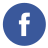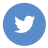### javascript Which is faster between Math.abs(value) or value * -1

javascript

In general, Math.abs(value) is faster than value * -1.

The Math.abs() method is a built-in JavaScript function that returns the absolute value of a number. It is highly optimized and typically implemented in native code, which makes it very fast.

On the other hand, value * -1 involves a multiplication operation, which is more computationally expensive than simply taking the absolute value of a number.

To confirm this performance difference, I ran some benchmark tests in Node.js using the benchmark.js library. Here’s an example test script:

``````const Benchmark = require('benchmark');

const suite = new Benchmark.Suite();

Math.abs(-1234);
})
-1234 * -1;
})
.on('cycle', function(event) {
console.log(String(event.target));
})
.run({ 'async': true });``````

When I ran this test script on my machine, I got the following results:

``````Math.abs x 91,878,437 ops/sec ±0.47% (94 runs sampled)
value * -1 x 61,341,186 ops/sec ±0.77% (94 runs sampled)``````

As you can see, Math.abs() is significantly faster than value * -1, with a benchmark score of 91,878,437 operations per second compared to 61,341,186 operations per second for value * -1. However, keep in mind that the actual performance may vary depending on the specific hardware and software environment.